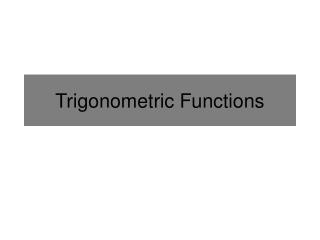Download PresentationTrigonometric Functions

# Trigonometric Functions

Download Presentation## Trigonometric Functions

- - - - - - - - - - - - - - - - - - - - - - - - - - - E N D - - - - - - - - - - - - - - - - - - - - - - - - - - -
##### Presentation Transcript

1. Trigonometric Functions

2. Trigonometric Identities The following identities need to be memorized: Pythagorean Identities Standard/Reciprocal Identities Double-Angle Identities

3. Measure of an Angle 1 The measure (counter clockwise) of an angle is determined by the amount of rotation from the initial side to the terminal side. -1 1 Initial Side Terminal Side -1

4. Reference Angle 1 The measure of a reference angle is the acute angle formed by the terminal side. and the x-axis. -1 1 Initial Side Terminal Side -1

5. 1 (cosӨ,sinӨ) Ө -1 1 -1 Coordinates on the Unit Circle This helps us find exact values for sine and cosine. Example: Find the coordinates for a 210°angle in the unit circle.

6. Exact Coordinates on the Unit Circle 1 90° 120° 60° 135° 45° These coordinates tell you the exact values of cosine and sine for 16 angles. 150° 30° They need to be memorized. 0° 180° -1 1 330° 210° 315° 225° 300° 240° 270° -1

7. Exact Coordinates on the Unit Circle NOTICE: Angles with the same reference angle have the similar coordinates. 1 π / 2 2π / 3 π / 3 3π / 4 π / 4 A majority of your work in calculus will be with radians, not degrees. 5π / 6 π / 6 π 0 -1 1 11π / 6 7π / 6 7π / 4 5π / 4 5π / 3 4π / 3 This needs to be memorized. 3π / 2 -1

8. Tricks of the Trade WARNING: Although shown, use these with great caution.

9. Trick #1: Special Right Triangles Use Special Right triangles to remember the first quadrant. 45°-45°-90° OR Isosceles Right 60°or π/ 3 1 1 or π / 6 30° 45° 45° or π/ 4 or π/ 4 30°- 60°- 90°

10. Trick #2: Finding a Reference Angle in Radians On the left are 3 reference angles that we know exact trig values for. To find the reference angle for angles not in the 1st quadrant (the angles at right), ignore the integer in the numerator. Bonus: Multiply the number in the numerator by the degree to find the angle’s quadrant.

11. Example Find the reference angle and quadrant of the following: Or 45º

12. Trick #3: Stewart’s Table for the First Quadrant Find the reference angle’s value, then the quadrant to figure out the sign (+/-) Square root # goes up 1 Reverse sine

13. Trick #4: Deciding whether a Trigonometric Function is Positive 1 Just Sine (and cosecant) All S A STUDENTS ALL -1 1 TAKE CALCULUS T C Just Tangent (and cotangent) Just Cosine (and secant) -1

14. Example 1 Find the exact value of the following: Thought process The only thing required for a correct answer (unless the question says explain)

15. Example 2 Remember: There are typically 2 answers. Solve: Isolate the Trig Function What angles make the sine function 0.5? When does 4x equal our two angles? when What other angles make the sine function 0.5? Sine is cyclic, how often does it repeat?

16. How Well do you know Trig?

17. How Well do you know Trig?

18. How Well do you know Trig?

19. How Well do you know Trig?

20. How Well do you know Trig?